Published: 25 April 2019

# Comparison of the performance of friction pendulums with uniform and variable radii

Cristian-Tatian Malin1
Dorian Nedelcu2
Gilbert-Rainer Gillich3
1, 2, 3, 4Eftimie Murgu University of Resita, Resita, Romania
5Politehnica University of Timisoara, Timisoara, Romania
Corresponding Author:
Dorian Nedelcu
Views 165

#### Abstract

The paper presents research done by means of numerical simulation on a rigid structure isolated with friction pendulums. To this aim, we design friction pendulums which differ by the shape and dimension of the cylindrical sliding surface, respectively by the friction coefficients. Our target was to find out how the structure responds to a given excitation when the structure is equipped with diverse friction pendulums. A sinusoidal excitation with the frequency of 1 Hz is applied and the response in terms of displacements is captured. We found that the frequency of the structure does not change with the FP radius but the amplitude of the displacement is strongly dependent on this parameter. Because the circular and elliptical sections of the FP provide the structure with different natural frequencies, the resonance is achieved at other radii.

## 1. Introduction

Base isolation for the protection of structures during earthquakes became an important issue in the last decades because the trepidations that manifest on a large area around the epicenter cause damages to the infrastructure and can result in human losses [1-3]. Advancements in seismic isolation were made in the last decades, now being available solutions that ensure good results in reducing the effects of the earthquakes [4-6]. Inserting elastomeric elements between the ground and the foundation of the structure had become a common solution . An analysis of the behavior of laminated elastic elements consisting of rubber and steel sheets is made in . The effect of a supplementary added lead core to the laminated steel-rubber bearings is investigated in . A highly nonlinear effect is obtained if an elastomeric bearing is combined with a lead-rubber bearing resulting in the so-called hybrid lead rubber bearing . Models of such rubber bearings describing their specific behavior are available [11-13]. To develop reliable analytical and numerical models, it is important to know the non-linear behavior of the elastic layer constituted by rubber or neoprene .

A particular seismic isolation device that is based on the dissipation of the earthquake-induced energy by friction is now used for several decades . This device, known as the friction pendulum (FP), has proved its maturity. However, a lot of research concerns the improvement of controlling the response of the isolated structure. To this aim, pendulums with more than one sliding surfaces are now developed . A new trend in the design of FPs is the use of adaptive sliding surfaces such as those based on polynomial curves [17, 18].

Our previous studies focused on finding the response of structures isolated with FPs that have different radii, friction coefficients and are subjected to harmonic or random excitation . Experiments are also made on a special shaking table developed in our laboratory . The study we describe in the following aimed to find the influence of the curvature of the sliding surface on the response of the isolated structure under harmonic excitations.

## 2. Materials and methods

To obtain the response of a five-story rigid structure isolated with diverse FPs, we performed simulations with SolidWorks, in particular by using the Motion module. The isolated structure’s 3D geometrical model, described in Fig. 1, is similar to that presented in . The assembly consists of three parts: (1) the rigid structure; (2) the base plate used as a reference; (3) the shaking plate simulating the horizontal trepidation of the earth. The latter component includes also the FPs.

The rigid structure is made of steel $L$ profile with the following dimensions of the cross-section: size $w=$ 25 mm and the thickness $t=$ 1 mm. The main dimensions of the assembly are indicated in Fig. 1.

Simulations are made in the following conditions:

a) The base plate, which is set as a reference, is fixed.

b) The shaking plate simulating the horizontal trepidation of the earth is harmonically moved in the $X$ direction. This is made with the help of the Linear Motor, a feature of SolidWorks Motion module. It imposes a Max Displacement $A=$ 10 mm by a Frequency ${f}_{exc}=$ 1 Hz.

c) The gravitational acceleration is set by default as $g=$ 9806.65 mm/s2 and is oriented in the $Y$ direction.

d) The time for this study is imposed as 30 seconds.

In this study, the mentioned parameters are maintained unchanged, but the radii of the sliding surfaces are modified by each simulation. However, for all simulations these are modeled as an extrusion applied to the shaking plate.

Fig. 1The assembly modeled in SolidWorks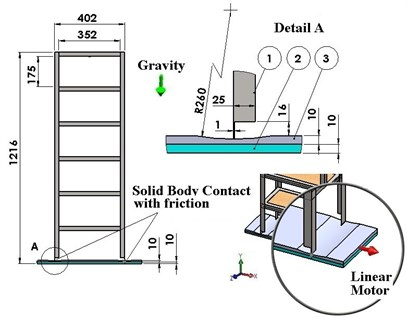For the first set of simulations, the base of the cylinder used for extrusion is a circle, the radius being modified in the range $R=$ 110/960 mm by a 50 mm pitch. The depth of the extrusion is 4 mm, therefore the minimum thickness of the shaking plate under the pendulum is 6 mm. A suggestive image showing all radii used to create of the FP is given in Fig. 2.

Table 1Contact condition based on friction coefficients

 Contact case Components Contact type ${\mu }_{D}$ [–] ${\nu }_{D}$ [mm/s2] ${\mu }_{S}$[–] ${\nu }_{S}$ [mm/s2] 1 Structure Steel (dry) 0.25 10.16 0.3 0.1 Shaking plate Steel (dry) 2 Structure Steel (greasy) 0.05 10.16 0.08 0.1 Shaking plate Steel (greasy) 3 Structure Teflon 0.03 10.16 0.05 0.1 Shaking plate Steel (greasy)

The contact condition between the structure and the sliding surface is bounded contact with friction, the friction coefficient $\mu$ being dependent of the chosen materials. Note that the friction coefficient depend on the relative speed beteen the two components in contact. We took the values for the first two cases in Table 1 from the SolidWorks library, while for the third case we defined the values to achieve a lower damping.

Fig. 2The extrusions made with a circular shape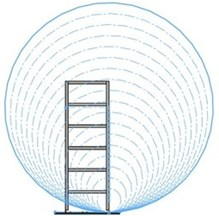a)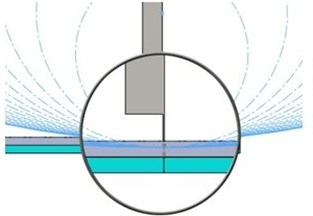b)

In the absence of friction between the structure and the sliding surface, the natural frequency ${f}_{n}$ of a rigid structure isolated with a FP is found from the mathematical relation:

1
${f}_{n}=2\pi \sqrt{\frac{R}{g}}.$

From Eq. (1) clearly results that ${f}_{n}$ is independent of the weight of the structure. If subjected to a harmonic excitation ${f}_{exc}$, the structure oscillates in respect to the two mentioned frequencies. When the relation between the two frequencies is ${f}_{n}<{f}_{exc}$, the frequency of the structure is the frequency of the excitation. If ${f}_{n}>{f}_{exc}$, the system maintain its natural frequency no matter how big the frequency of the excitation is. When the two amplitudes achieve close values, the amplitude of the oscillation increases dramatically, and for equal frequencies the resonance is attained. The amplitude resulted in resonance depends on the friction coefficient; the smaller the friction coefficient, the bigger the amplitude is.

For the second set of simulations, we use for extrusion cylinders that have ellipses at the base. The vertically radius of the ellipse is always ${R}_{V}=$ 960 mm, while the horizontally radius is modified in the range ${R}_{H}=$ 110/960 mm by a 50 mm pitch, as shown in Fig. 3.

Fig. 3The extrusions made with an elliptical shape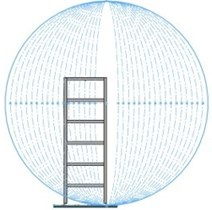a)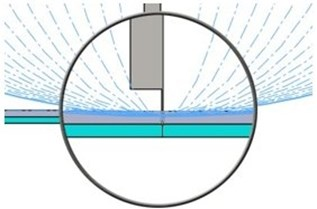b)

Because the slope of the sliding surface is significantly bigger for the case ellipse 110-960 than for the case radius 110, we expect in the case of the sliding surface having constructed with an elliptical cylinder to get no relative displacement between the structure and the shaking plate. We also expect to achieve the resonance at higher values of the horizontal radius of the ellipse as the radius of the cylinder.

It is no relation presented in the literature for determining the natural frequency of the elliptical FP, thus the behavior in terms of frequencies and amplitudes of the oscillation of the isolated structure cannot be predicted. By the simulations performed with elliptical FPs, we intend to clarify this aspect.

## 3. Results and discussions

We present in Fig. 4 the signals in the time domain and the frequency domain (by FFT representation) for three typical behaviors of both the FPs. In the left column in this figure we represented the analysis for a pre-resonance behavior, in the central column the behavior in resonance and in the right column the behavior in post-resonance. The friction coefficient is chosen for the contact case 1 (steel dry – steel dry).

Fig. 4Response signal captured from the isolated structure for different friction pendulum radii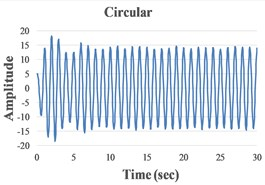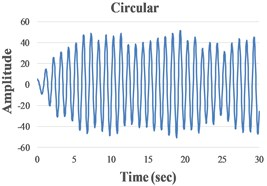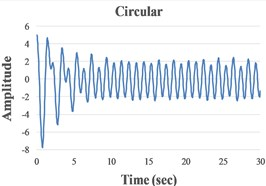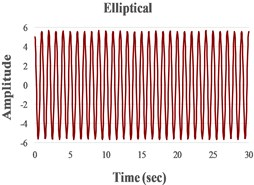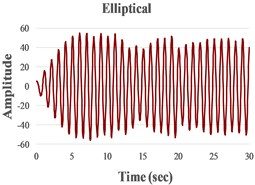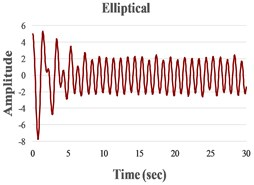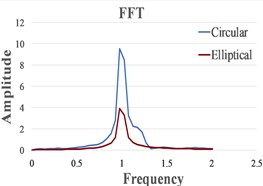a)$R={R}_{H}=$ 160 mm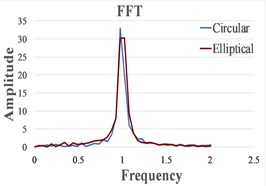b)$R=$ 260 mm, ${R}_{H}=$ 460 mm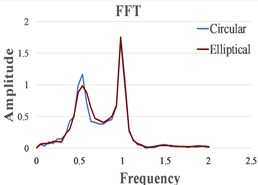c)$R={R}_{H}=$ 910 mm

In Fig. 5 we offer a comprehensive image on the behavior of the structures when it is isolated with FPs that have all considered radii and both circular and elliptic cylindrical shapes. In this figure, the minim and maxim values of the structure’s displacements in the $X$ direction are indicated in order to show for which horizontal radii the resonance is achieved.

One can observe in Fig. 5 that, as expected, the resonance is achieved for smaller values of $R$ in comparison with ${R}_{H}$. This determines a bigger pre-resonance domain for the elliptical FP, while the post-resonance is earlier achieved by the FP constructed with a circular cylindrical sliding surface. The magnitudes of the curves represented in Fig. 5 are quite similar for similar friction coefficients. Because the magnitudes are extracted from the signals by involving the FFT, just coarse values are obtained. To precisely estimate the magnitudes an advanced signal processing algorithm is requested .

Fig. 5Maximum and minimum displacement amplitudes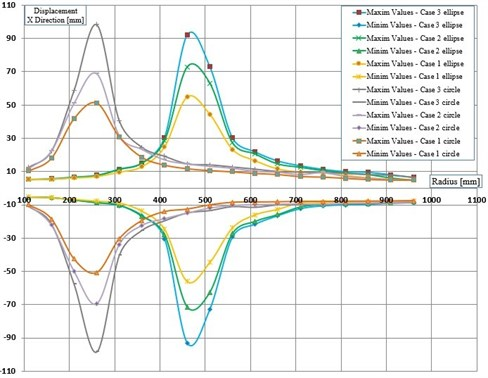## 4. Conclusions

The paper presents a comparison between the responses of a structure isolated by different types of friction pendulums. We found that it is possible to control the occurrence of resonance by changing the horizontal radius of the ellipse, therefore it is possible to design the FP in order to work in pre- or post-resonance depending on the parameters (frequency and amplitude) expected for the ground excitation. For both set of pendulums we concluded that the best isolation of the structure is achieved when the natural frequency of the pendulum is at least 1.5 times lower than the ground excitation. We also found that the friction coefficient has the same influence on the amplitudes of the structure’s response it the FP has the same natural frequency. This is best visualized at resonance, the curves in Fig. 5 achieving approximately the same magnitude. Our next research will focus on finding a relationship to express the natural frequency of elliptical friction pendulums.

#### Cited by

Dynamic Equilibrium Equations in Unified Mechanics Theory
A Multibody Inertial Propulsion Drive with Symmetrically Placed Balls Rotating on Eccentric Trajectories
Displacement and velocity estimation of the earthquake response signals measured with accelerometers